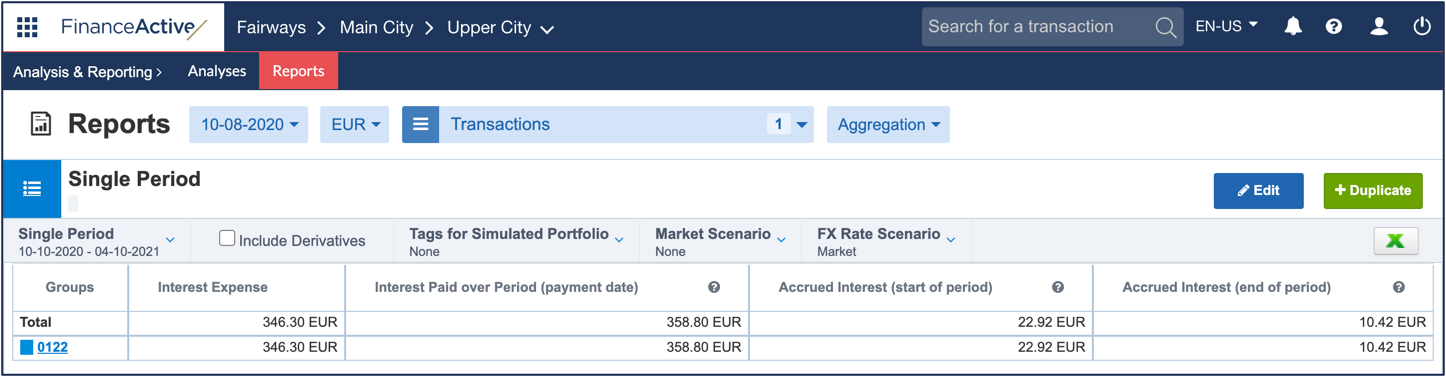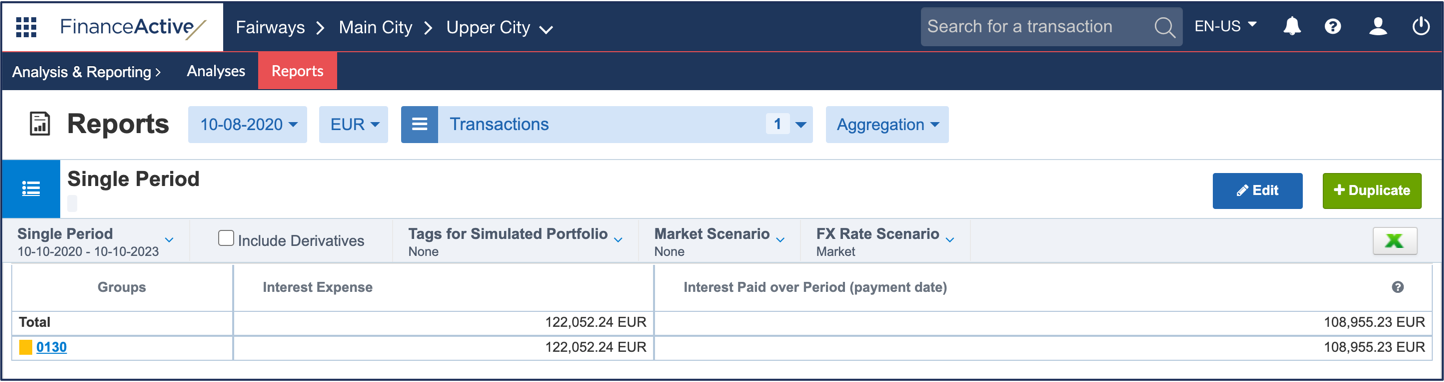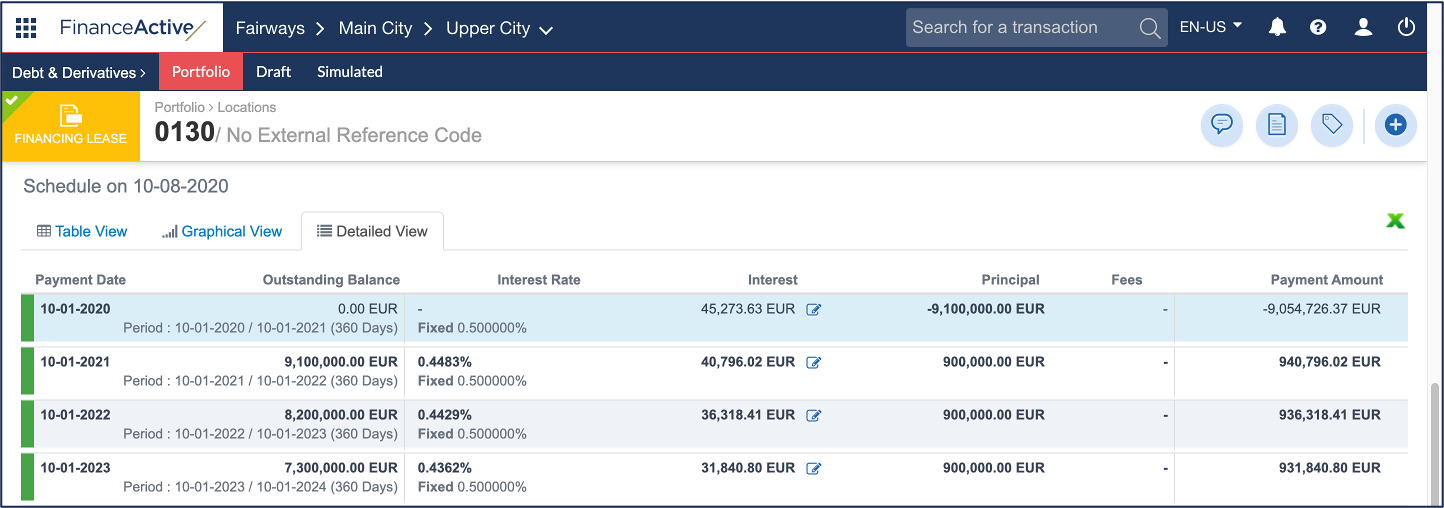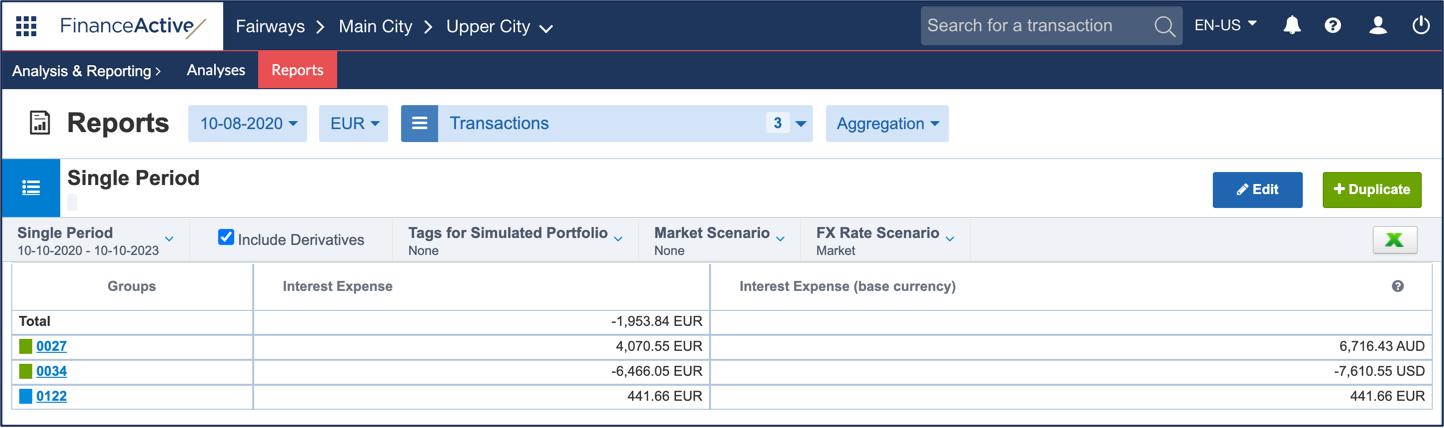# Interest Expense

In Fairways Debt, the interest expense is the interest charged during the analysis period.

## Periodic / Single Period Reports

Interest Expense

Interest expense with all amounts converted in the currency applied to the report:

For example, the interest expense of 0122 is:

= Interest Paid over Period (payment date) - Accrued Interest (start of period) + Accrued Interest (end of period)

= 358,80 - 22,92 + 10,42

= 346,30The computation for leases in advance differs from the standard formula:

Interest Paid over Period (payment date) + Decreased interest (start of period) - Decreased interest (end of period)

= Interest Paid over Period (payment date) + Interest / Number of days of the schedule period * Number of days between the end date of the schedule period and the start date of the report period - Interest / Number of days of the schedule period * Number of days between the end date of the schedule period and the end date of the report period

Note: Prorata interest over partial periods (decreased interest) are defined using the actual settings of the relevant transaction.

For example, the interest expense of 0130 is:

= Interest Paid over Period (payment date) + Decreased interest (start of period) - Decreased interest (end of period)

= 108 955,23 + 44 141,79 - 31 044,78

= 122 052,24Decreased interest (start of period)

= Interest / Number of days of the schedule period * Number of days between the end date of the schedule period and the start date of the report period

= 45 273,63 / 360 * 351

= 44 141,79

• The report start date is 10 October 2020, which corresponds to the 1 October 2020 - 1 October 2021 period of the transaction.
• The interest of that period is EUR 45 273,63.
• The period lasts 360 days.
• The transaction period ends on 1 October 2021, 351 days apart from the report start date on 10 October 2020.Decreased interest (end of period)

= Interest / Number of days of the schedule period * Number of days between the end date of the schedule period and the end date of the report period

= 31 840,80 / 360 * 351

= 31 044,78

• The report end date is 10 October 2023, which corresponds to the 1 October 2023 - 1 October 2024 period of the transaction.
• The interest of that period is EUR 31 840,80.
• The period lasts 360 days.
• The transaction period ends on 1 October 2024, 351 days apart from the report end date on 10 October 2023.

Interest Expense (base currency)

Interest expense with all amounts in the currencies initially applied to the transactions.

In this example, we can see the currencies of 0027 and 0034 are different from the report currency (AUD and USD vs. EUR).